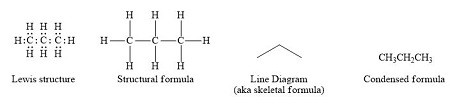# Draw the condensed formulas for each of the following compounds. a. Methoxyethene b....

## Question:

Draw the condensed formulas for each of the following compounds.

a. Methoxyethene

b. Triethylamine

c. 1-propanol

d. bromochlorofluoroiodomethane

## Condensed Formula:

The molecular formula which is represented in the form without lines as a bond, and just shows a symbolic representation of atoms is known as condensed form. It is written in a straight line without branching. The atom-like C, N, O, S, X (=Cl, Br, I) and H are written in the symbolic form with the number as a subscript to represent the number of atoms in the molecule. For example, methane can be written as {eq}C{H_4} {/eq}